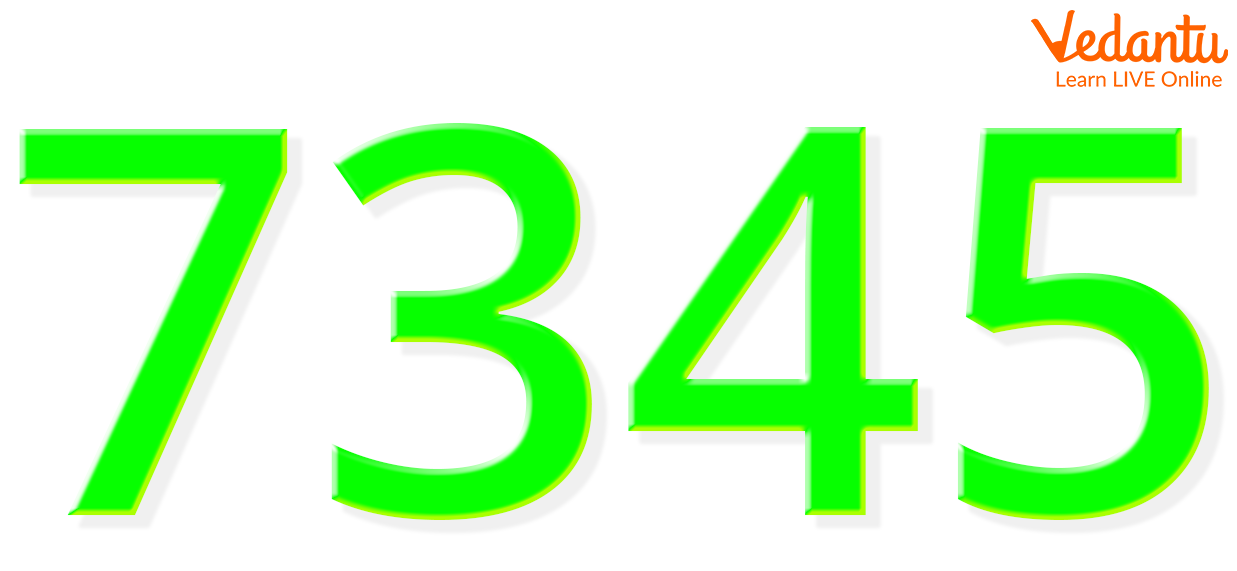Courses
Courses for Kids
Free study material
Offline Centres
More

# Where is the Ones Place?Last updated date: 30th Nov 2023
Total views: 101.4k
Views today: 2.01k## Introduction to Ones Place

In general, a number's place value indicates the value of a certain digit based on that digit's position inside the number, among other factors. The one's place or unit's place refers to the location within a number. The number itself is the value of the digit in the one's position.

### What is Place Value?

The term "place-value" is typically used to describe the importance of a digit in a given number based on the location of that digit inside that number. According to the number's digits, the locations in a number are ordered starting with the rightmost digit, such as the one's place, tens place, hundreds place, and so on. For instance, since 6 is in the tens place in the number 468, its place value is 60.

### What is One’s Place?

One's place is defined as the first digit from the left or the rightmost position of a number. Any number's rightmost digits are referred to as the number's "one's position." The value of the digit in one's position is the same as the value of the digit itself in terms of place value. Consider the number 342, for instance. The rightmost number is 2, and its place value is 2 itself.

### How to Identify One’s Place?

With the exception of integers that contain decimals, the rightmost position is regarded as the one's place. In the case of decimals, the one's place is the position immediately to the right of the decimal point. For single-digit integers, the number itself takes the place of one.Example to Identify One’s Place

In the image above, the rightmost digit is 5. Hence, the number in the one’s place is 5

### Solved Examples :

1. Identify the digit in the one’s place in the number 7,45,67.

Solution: Here, in the number 7,45,67 the rightmost digit is 7. Hence, the digit in one's place is 7.

1. Identify the digit in the one’s place in the number 72.

Solution: Here, in the number 72 the rightmost digit is 2. Hence, the digit in one's place is 2.

1. Identify the digit in the one’s place in the number 94.33.

Solution: Here, in the number 94.33 the rightmost digit before the decimal point is 4. Hence, the digit in one's place is 4

1. Identify the digit in the one’s place in the number 10,000.

Solution: Here, in the number 10,000 the rightmost digit is 0. Hence, the digit in one's place is 0.

1. Identify the digit in the one’s place in the number 468.23.

Solution: Here, in the number 468.23 the rightmost digit before the decimal point is 8. Hence, the digit in one's place is 8.

### Conclusion

As a result, the place value is used to determine a number's digit value. The one's place is at the number's rightmost position. Therefore, it is assumed that the digit in the number's rightmost position is the one whose value equals the number itself.

## FAQs on Where is the Ones Place?

1. Can 0 be considered to be a digit in one's place?

Yes, 0 can occupy the position in the one’s place except for single digit numbers as in single digit numbers 0 will hold no value.

2. Which is the one's place position in case of recurring numbers?

The position occupied by a digit preceding the decimal point is the one’s place in that number.

3. What is the value of the digit in one’s place of 670?

The digit in one’s place of 670 is 0. Therefore, its place value is also 0.

4. Is 0 considered a valid digit to occupy one’s place in a decimal number?

Yes, 0 is a valid number if placed in the one’s place of a decimal number.

5. Can there be a number with no digit in one's place?

No, there cannot be such a number without one's place.